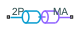# Interface (2P-MA)

Two-phase fluid and moist air network interface

• Library:
• Simscape / Fluids / Fluid Network Interfaces

•## Description

The Interface (2P-MA) block represents a flow connection between a two-phase fluid network and moist air network. Pressure, temperature, and mass flow rate are equal at the interface. The block does not transfer the fluid properties of each fluid network across the interface. When moist air leaves port MA, the block assigns the values of the Interface relative humidity and Interface trace gas mass fraction parameters to the signal. However, when moist air enters port MA, the block retains the upstream values.

You can choose whether to maintain constant moisture and trace gas amounts at the interface or to specify them using the W and TG ports respectively. The fluid at the 2P port must remain superheated. You can use the Two-phase fluid superheated vapor check parameter to choose what action the block performs if the fluid does not meet this condition.

### Equations

The block conserves mass such that

`${\stackrel{˙}{m}}_{2P}+{\stackrel{˙}{m}}_{MA}=0,$`

where:

• 2P is the mass flow rate into port 2P.

• MA is the mass flow rate into port MA.

The block maintains equal port pressures and temperatures such that

`$\begin{array}{l}{p}_{2P}={p}_{MA}\\ {T}_{2P}={T}_{MA}\end{array}$`

The block calculates the energy balance over the interface as

`${\Phi }_{2P}+{\Phi }_{MA}={\stackrel{˙}{m}}_{2P}\left({h}_{2P}+\frac{{w}_{2P}^{2}}{2}\right)+{\stackrel{˙}{m}}_{MA}\left({h}_{MA}+\frac{{w}_{MA}^{2}}{2}\right),$`

where:

• Φ2P is the energy flow rate into port 2P.

• ΦMA is the energy flow rate into port MA.

• h2P is the specific enthalpy at port 2P.

• hMA is the specific enthalpy at port MA.

• v2P is the specific volume at port 2P.

• vMA is the specific volume at port MA.

• w2P is the flow velocity at port 2P.

• wMA is the flow velocity at port MA.

For a given cross-sectional area, S, the block defines w2P as

`${w}_{2P}=\frac{{\stackrel{˙}{m}}_{2P}{v}_{2P}}{S}.$`

and wMA as

`${w}_{MA}=\frac{{\stackrel{˙}{m}}_{MA}{v}_{MA}}{S},$`

where

`${v}_{MA}=\frac{{R}_{I}{T}_{MA}}{{p}_{MA}}.$`

You specify S using the Cross-sectional area at ports 2P and MA parameter. The block computes the specific gas constant of the interface mixture, RI, as a function of the fluid on either side such that

`${R}_{I}=f\left({R}_{A},{R}_{W},{R}_{G},{x}_{WI},{x}_{GI}\right),$`

where:

• RA is the dry air specific gas constant.

• RW is the water vapor specific gas constant.

• RG is the trace gas specific gas constant.

• xWI is the interface specific humidity. When the flow exits port MA, the block assigns this value based on the value of the Interface relative humidity parameter or the input that you supply at port W.

• xGI is the interface trace gas mass fraction. When the flow exits port MA, the block assigns this value based on the value of the Interface trace gas mass fraction parameter or the input that you supply at port TG.

## Ports

### Input

expand all

Physical signal port associated with the moisture content at the interface.

#### Dependencies

To enable this port, set Moisture and trace gas specification to `Controlled`.

Physical signal port associated with the trace gas at the interface.

#### Dependencies

To enable this port, set Moisture and trace gas specification to `Controlled`.

### Conserving

expand all

Two-phase fluid conserving port associated with the two-phase fluid at the interface.

Moist air conserving port associated with the moist air at the interface.

## Parameters

expand all

Option to maintain a constant interface or to specify the moisture and trace gas amounts via physical signal ports. When you select `Controlled`, the block exposes the W and TG ports.

Whether to calculate moisture using relative humidity, specific humidity, mole fraction, or humidity ratio.

Relative humidity at the interface. The block uses this value to calculate the moisture.

#### Dependencies

To enable this parameter, set Interface type to `Constant` and Moisture specification to ```Relative humidity```.

Specific humidity at the interface. The block uses this value to calculate the moisture.

#### Dependencies

To enable this parameter, set Interface type to `Constant` and Moisture specification to ```Specific humidity```.

Water vapor mole fraction at the interface. The block uses this value to calculate the moisture.

#### Dependencies

To enable this parameter, set Interface type to `Constant` and Moisture specification to ```Mole fraction```.

Humidity ratio at the interface. The block uses this value to calculate the moisture.

#### Dependencies

To enable this parameter, set Interface type to `Constant` and Moisture specification to ```Humidity ratio```.

Whether to parameterize the trace gas at the interface using mass fraction or mole fraction.

Mass fraction of trace gas at the interface.

#### Dependencies

To enable this parameter, set Interface type to `Constant` and Interface trace gas specification to ```Mass fraction```.

Mole fraction of trace gas at the interface.

#### Dependencies

To enable this parameter, set Interface type to `Constant` and Interface trace gas specification to ```Mole fraction```.

Relative humidity at the point of saturation.

Cross-sectional area of the two-phase fluid and moist air ports.

Action to perform when the fluid at the interface does not meet superheated conditions.

Monitor or control invalid inputs. When you select ```Limit to valid values```, the block saturates the inputs within the bounds. You can choose to have the block issue a warning when it limits the inputs by selecting ```Warn and limit to valid values```, or you can stop the simulation when the block receives invalid inputs by selecting `Error`.

#### Dependencies

To enable this parameter, set Interface type to `Controlled`.

## Version History

Introduced in R2022a